## why applyrule worked but not algsubs on this examp...

Asked by:

I never used applyrule before. Seems like a good command. I was trying to tell Maple to rewrite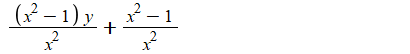as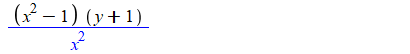I could not find one command to do it.  Collect does not work. The only way I figure to it, is by replacing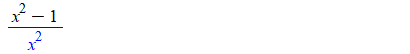by a new single unused symbol, and then use collect on the symbol, then use subs again to replace the symbol with orginal  expression. like this

```restart;
expr:=(x^2 - 1)/x^2*y + (x^2 - 1)/x^2;
r:=(x^2 - 1)/x^2; #this is what I want to collect
applyrule(r=Z,expr);
collect(%,Z);
subs(Z=r,%)
```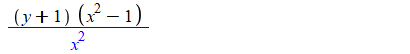In the above code, if I replace applyrule by algsubs, it does not work. Why?

```restart;
expr:=(x^2 - 1)/x^2*y + (x^2 - 1)/x^2;
r:=(x^2 - 1)/x^2;
algsubs(r=Z,expr);
```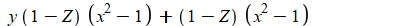You see, it did not replace (x^2-1)/x by Z, like applyrule does:

```restart;
expr:=(x^2 - 1)/x^2*y + (x^2 - 1)/x^2;
r:=(x^2 - 1)/x^2;
applyrule(r=Z,expr);
```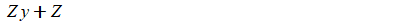I will learn applyrule now more to do this. I was just wondering why algsubs failed, and if applyrule is the better tool to do this. Or may be there is a better way to do this whole thing?

Maple 2019.1

## How to update a matrix in a proc?...

Asked by:

I expect this proc to return a matrix of all ones but it returns a matrix of all zeros.  Why does it do that?

```doit := proc()
local i, j, M;
M := Matrix(3,3);
for i from 1 to 3 do
for j from 1 to 3 do
M[i][j] := 1;
end do;
end do;
return M;
end proc:

doit();
[0    0    0]
[           ]
[0    0    0]
[           ]
[0    0    0]
```

## How to Move Images...

Asked by:

I am putting pictures into Maple 2019 Document to facilitate/document of analysis being done.  Would very much like to move pictures/images around document?  Once I place them, I can resize them, but I cannot seem to move them.  This is very restrictive.

Is there a solution?

Thank you in advance for your help.

## Parsing mistake in MAPLE...

Asked by:

In equation(19), the value for F = 14.616.. is wrong. It was produced by a bult execution of the whole program. However, executing only equation(19) in one step results in the correct answer F=24.665. The values for SSE,SSR, which are 1 x 1 matrices, are correct. Indices(1x1) provide access to the elements as constants so that arithmetic operations can be performed.

The correct result follows an execution at eqn(19) as a stand alone. An incorrect result follows execution of the entire program.

restart:

interface(rtablesize = 50); with(LinearAlgebra); with(plots); with(Statistics); with(ArrayTools);

Y := Matrix(12, 1, [2, 3, 2, 7, 6, 8, 10, 7, 8, 12, 11, 14]); n := ArrayNumElems(Y);

This is the data, with n rows and one column.

[2 ]

[  ]

[3 ]

[  ]

[2 ]

[  ]

[7 ]

[  ]

[6 ]

[  ]

[8 ]

Y := [  ]



[  ]

[7 ]

[  ]

[8 ]

[  ]



[  ]



[  ]



n := 12

type(Y, Matrix);

true

X := Matrix(12, 3, [1, 0, 2, 1, 2, 6, 1, 2, 7, 1, 2, 5, 1, 4, 9, 1, 4, 8, 1, 4, 7, 1, 6, 10, 1, 6, 11, 1, 6, 9, 1, 8, 15, 1, 8, 13]); k := NumElems(X)/n;

[1  0  2 ]

[        ]

[1  2  6 ]

[        ]

[1  2  7 ]

[        ]

[1  2  5 ]

[        ]

[1  4  9 ]

[        ]

[1  4  8 ]

X := [        ]

[1  4  7 ]

[        ]

[1  6  10]

[        ]

[1  6  11]

[        ]

[1  6  9 ]

[        ]

[1  8  15]

[        ]

[1  8  13]

k := 3

Xprimed := Transpose(X);

Calculate Multiple Linear Regression in x1, and x2

[1  1  1  1  1  1  1  1   1   1  1   1 ]

[                                      ]

Xprimed := [0  2  2  2  4  4  4  6   6   6  8   8 ]

[                                      ]

[2  6  7  5  9  8  7  10  11  9  15  13]

XprimedX := Xprimed . X;

[12   52   102 ]

[              ]

XprimedX := [52   296  536 ]

[              ]

[102  536  1004]

XprimedXinverse := MatrixInverse(XprimedX);

[   309          77              145 ]

[   ---          ---      uminus0--- ]

[   317          317             634 ]

[                                    ]

[    77          411             141 ]

XprimedXinverse := [   ---         ----      uminus0----]

[   317         2536             1268]

[                                    ]

[       145         141       53     ]

[uminus0---  uminus0----      ---    ]

[       634         1268      634    ]

XprimedY := Xprimed . Y;

[90 ]

[   ]

XprimedY := 

[   ]



Betahat := XprimedXinverse . XprimedY;

[   1704   ]

[   ----   ]

[   317    ]

[          ]

[   3819   ]

Betahat := [   ----   ]

[   1268   ]

[          ]

[       815]

[uminus0---]

[       634]

Betahat := convert(Betahat, float);

[    5.375394322     ]

[                    ]

Betahat := [    3.011829653     ]

[                    ]

[&uminus0;1.285488959]

We choose the Null Hypothesis that Y= β0+Β1*x1+Β2*x2+ϵ .

Performing multiple linear regression leads to the best fit linear equation:

Y= 5.38+3.01*x1-1.29x2

fit := plot3d(5.38+3.01*X1-1.29*X2, X1 = 0 .. 8, X2 = 2 .. 15);

data := pointplot3d({[0, 2, 2], [2, 5, 7], [2, 6, 3], [2, 7, 2], [4, 7, 10], [4, 8, 8], [4, 9, 6], [6, 9, 12], [6, 10, 7], [6, 11, 8], [8, 13, 14], [8, 15, 11]}, axes = normal, color = red, symbol = soliddiamond, symbolsize = 40, scaling = unconstrained, title = `\` Data vs Best Fit ' `);

display({data, fit});

Comparison of Data and Best Fit

Yprimed := Transpose(Y); YprimedY := Yprimed . Y; BetahatPrime := Transpose(Betahat);

Yprimed := [2  3  2  7  6  8  10  7  8  12  11  14]

YprimedY := 

BetahatPrime := [5.375394322  3.011829653  &uminus0;1.285488959]

SSE := YprimedY-BetahatPrime . XprimedY; SampleVariance := (YprimedY-BetahatPrime . XprimedY)/(n-k-1);

SSE := [25.4589905220000]

SampleVariance := [3.18237381525000]

SSE (below) is a 1x1 vector which has been changed into a constant                                                  Covariance of β

`cov&beta;` := Typesetting[delayDotProduct](SSE(1, 1), XprimedXinverse, true);

cov&beta; := [

[24.8164923384795, 6.18404501638486, &uminus0;5.82263978815458],

[6.18404501638486, 4.12604302229574, &uminus0;2.83100762113723],

[&uminus0;5.82263978815458, &uminus0;2.83100762113723,

2.12827523291167]]

SSR := Transpose(Betahat) . Transpose(X) . Y-n*MeanY(1, 1)^2;

SSR := [139.541009478000]

SST := SSR+SSE; F := SSR(1, 1)*(n-k-1)/(k*SSE);

F is found in textbook eqn (8.5)

SST := [165.]

F := [14.6160295824160]

RsquaredNonCentered := Determinant(SSR)/Determinant(SST); R := sqrt(%);

RsquaredNonCentered := 0.845703087745454

R := 0.919621165342259

MeanY := Mean(Y);

MeanY := [7.50000000000000]

RsquaredCentered := `~`[`.`](Transpose(Betahat) . Transpose(X) . Y-n*MeanY(1, 1)^2, 1/(Yprimed . Y-n*MeanY(1, 1)^2));

RsquaredCentered := [0.845703087745454]

We note the same value for R -data not centered, and that for R-data which is centered.

Transpose(Betahat) . Transpose(X) . Y; MeanY(1, 1)^2; Yprimed . Y;

# Compare SSR, Mean of Y (data), and SST to results in the book.

[814.541009478000]

56.2500000000000



Table 8.2 on Page 189 of textbook

ANOVA for Overall Regression Test for Data in Table 7.1

________________________________________________________________________________________________________

Source           df                                                   SS                                                        MS                                                 F

_________________________________________________________________________________________________________

Include β1    2                                        139.5410                                    69.7705                                24.665

Error           9                                          25.4590                                      2.8288

Total                                             165.0000

_________________________________________________________________________________________

and from calculations using the defining equations in step(12) through step(13) and summarized in Step(19):

F := SSR(1, 1)*(n-k-1)/(k*SSE(1, 1)); SSE, SSR, SSR+SSE; Total := Yprimed . Y-n*MeanY(1, 1)^2;

F := 14.6160295824160

[25.4589905220000], [139.541009478000], [165.]

Total := [165.]

## Is it possible to instantiate all the attributes o...

Asked by:

Hi,

When creating a user random variable, I would like to instanciate some of its attributes, for instance ParentName.
But it seems that it's not always possible.

​​​​​​​Is it a Maple's limitation or am I not doing the things correctly ?
​​​​​​​
Example:

 > restart:
 > with(Statistics):
 > U := RandomVariable(Uniform(0, 1)):
 > interface(warnlevel=0):
 > A := attributes(U)(1)
 > AllAttributes := with(A);(2)
 > A:-ParentName(3)
 > # Define a user random variable v := Distribution(PDF = (z -> piecewise(0 <= t and t < 1, 1, 0))): V := RandomVariable(v): A := attributes(V); AllAttributes := with(A); A:-Conditions;(4)
 > # its definition can be augmented by adding some recognized attributes... # even if the result returned by Mean is strange v := Distribution(PDF = (z -> piecewise(0 <= t and t < 1, 1, 0)), 'Mean'=1/Pi, 'Median'=exp(-1)): V := RandomVariable(v): A := attributes(V); AllAttributes := with(A); [Median, Mean](V)(5)
 > # but not all the recognized attributes seem to be able to be instanciated: v := Distribution(PDF = (z -> piecewise(a <= t and t < b, 1/(b-a), 0)), 'Parameters'=[a, b]); v := Distribution(PDF = (z -> piecewise(a <= t and t < b, 1/(b-a), 0)), 'ParentNames'=MyDistribution);
 >

Download Attributes.mw

## how to plot different values of Mh...

Asked by:

How to get a plot for different values of Mh.

like Mh=[1 2 3 4]

Code:

restart;
with(DEtools,odeadvisor);

m:=10;H:=1;Mh:=1;b:=0.02; a:=0.05;V:=array(0..m); V:=1-exp(-t);

for k from 1 to m do

if k=1 then chi:=0;

chi:=1;

fi;

p:=0;

for j from 0 to k-1 do

p:=p+(V[k-1-j]*diff(V[j],t\$2)-diff(V[k-1-j],t)*diff(V[j],t)-a*(2*diff(V[k-1-j],t)*diff(V[j],t\$3)-diff(V[k-1-j],t\$2)*diff(V[j],t\$2)-V[k-1-j]*diff(V[j],t\$4)));  od;

p:=(p+diff(V[k-1],t\$3)-b*(diff(V[k-1],t\$2)+t*diff(V[k-1],t\$3))-Mh*diff(V[k-1],t))*h*H;

p:=factor(p);

V[k]:=(-int(p,t)+0.5*exp(t)*int(exp(-t)*p,t)+0.5*exp(-t)*int(exp(t)*p,t)+chi*V[k-1]+C1+C3*exp(-t));

v:=unapply(V[k],t);

V[k]:=frontend(expand,[V[k]]);  V[k]:=subs(C3=solve(eval(subs(t=0,diff(V[k],t))),C3),V[k]); V[k]:=frontend(expand,[V[k]]);

V[k]:=subs(C1=solve(eval(subs(t=0,-V[k]-diff(V[k],t))),C1),V[k]);

od:

appr:=0;

for k from 0 to m do

appr:=appr+V[k];

od:

u_appr:=unapply(appr,(h,t)):

u_appr_1:=unapply(diff(u_appr(h,t),t),(h,t)):

evalf(u_appr_1(-0.4,t)):

with(plots);

plot([u_appr_1(-0.4,t)],t=0..4,0..1.2,color=[black],axes=frame):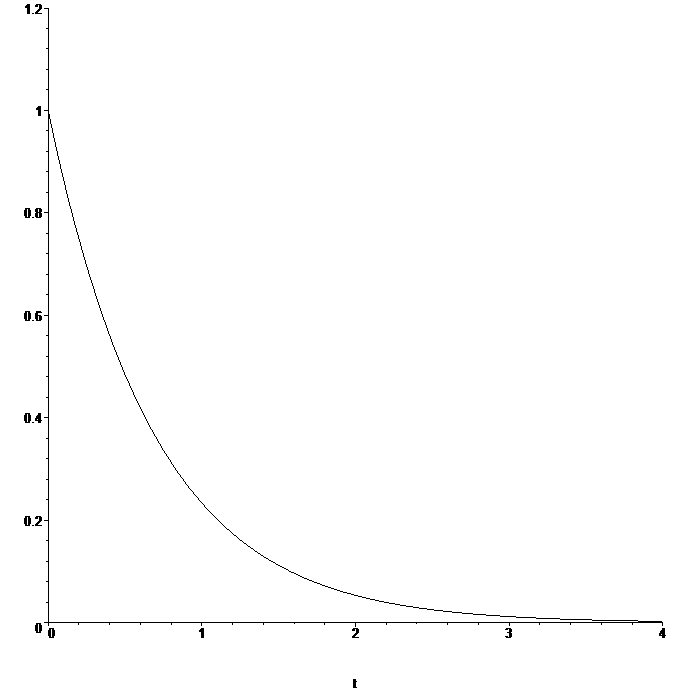this plot for Mh=1:

## Why is the result not evaluated?...

Asked by:

Hi,

How do I change the definition of g to get the result f(1, 2)(x) = 2*t+3 ?

f := a*x+b;
g := (a,b) -> x -> f;
g(a, b)(x);   # answer a*t+b
g(2, 3)(x);   # answer a*t+b

Thanks in advance

## How to modify an differential equation which passe...

Asked by:

Hey, I need to modify a differential equation passed to a procedure. My problem is that this code works fine:

```restart;
ode := diff(y(x), x) = y(x):
y := unapply(exp(x), x):
ode;
exp(x) = exp(x)
```

But this doesn't:

```restart;
ode := diff(y(x), x) = y(x);
p1 := proc (eq)
local y;
y := unapply(exp(x), x);
eq
end proc:
p1(ode);
d
--- y(x) = y(x)
dx
```

How to solve this problem?

## Command support large number...

Asked by:

May I know any command that able to support the large number even up to 2000 bits?

## Error, (in type/algext) too many levels of recursi...

Asked by:

I made a typo below, I did not mean to put [...] around the solution. But why Maple throws an error on this only?

```restart;
ode:=diff(y(x),x) = exp(x)*sin(x)+y(x)*cot(x);
my_sol:=[sin(x)*(exp(x)+_C1)];
odetest( y(x)=my_sol,ode) assuming x::real
```

Error, (in type/algext) too many levels of recursion

But on other ODE's, it works

```restart;
ode:=diff(y(x),x) = y(x);
my_sol:=[_C1*exp(x)];
odetest( y(x)=my_sol,ode) assuming x::real
```



I'll correct my type and remove the []. But the question is, should Maple have thrown an error? And why only on this one? Would this be considered a bug?

Removing the [] from the first example above, the error is gone and 0 is returned.

Maple 2019.1, Physics V 395 on windows 10

## How to use ssystem and friends...

Asked by:

I am getting my feet wet with Maple's system calls, like ssystem. I am considering to use Maple to run something like a Python script for certain numeric calculations, based on Maple-generated results. I am using Mac OS X (macOS for newer versions).

Maple's Help gives the basics and that works. For the specific Python example; I can run a simple script by using

ssystem("python -c A=2;print(A)"); # \n instead of ; works also.

Presumably I can do this with longer scrpts as well.

However, it appears that, when creating the process, Maple does not setup the environment the user (me) gets through initialization files like .profile, .cshrc or the like. As a result even a simple "python -c import sys" fails; presumably because the environment where Python looks for the module isn't setup. I tried

ssystem("source .cshrc");

but that fails as well. Trying to be clever and invoking a login shell (ssystem("tcsh -lc <command>");) also leads nowhere.

The Help page does say that ssystem() does not give access to all shell commands; is there somewhere documentation that says what works and what is not supported?

I am aware that Maple2018 and newer have more support in form of the Python package, but right now I need my code to be backward compatible to Maple2015 so this new package does not solve my issue for the older installation I am also using. Also, Python is not necessarily the only language I want to use.

Any suggestions would be welcome.

Mac Dude

## how to apply two for loops ...

Asked by:

How to apply two for loops to solve ode problem.

code:

restart; with(plots); fcns := {T(eta), f(eta)};
m := .5; bet := 1; na := 1/6; N := 5;
eq1 := (diff(f(eta), `\$`(eta, 3)))*pr+m-m*(diff(f(eta), `\$`(eta, 1)))+((m+1)*(1/2))*(diff(f(eta), `\$`(eta, 2)))*f(eta) = 0;
eq2 := diff(T(eta), `\$`(eta, 2))+((m+1)*(1/2))*(diff(T(eta), `\$`(eta, 1)))*f(eta) = 0;
bc := f(0) = 0, (D(f))(0) = 0, (D(f))(N) = 1, (D(T))(0) = -bi*(1-T(0)), T(N) = 0;
bi:= [seq(1..4,0.1)];  NN := nops(bi);
pr:=[seq(1..2,0.1)];  NN1 := nops(pr);
for i  from 1 to NN do
for j from 1 to NN1 do

R := dsolve(eval({bc, eq1,eq2}, bi[i],pr[j]), fcns, type = numeric, method = bvp[midrich], maxmesh=2400):
X1||[i,j]:=rhs(-R(0)):
end do:
end do:

Have a good day.

## Error while animation in 2d...

Asked by:

B1 := plots:-animate(plots:-pointplot, [[seq(X(kk, j), j = 1 .. 10)], [seq(Y(kk, j), j = 1 .. 10)]], kk = [seq(1 .. 50)], symbolsize = 25, symbol = solidcircle, colorscheme = ["valuesplit"])

gives error

Error, unsupported type of index, kk

Here X and Y are 50*10 matrices

## How to restrict matrix elements to whole numbers...

Asked by:

A minor question ....

Does anybody know how to format a matrix so all elements are presented in whole numbers?

It's more a niggle than anything .. I want to retain decimals in my worksheet but wish only to have the matrix display integer values.

Thank you!

## Generate large primes commands...

Asked by:

May I know which commands able to generate large prime up to 2000 bits in maple?

﻿